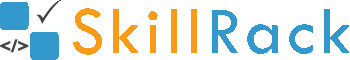ProgramID- 1617SKILLRACK
Printing N terms in Fibonacci series

The program must accept an integer N as the input. The program must print the first N terms in the fibonacci series as the output.

Boundary Condition(s):
3 <= N <= 30

Input Format:
The first line contains N.

Output Format:
The first line contains N integers representing the first N terms in the fibonacci series separated by a space.

Example Input/Output 1:
Input:
10

Output:
0 1 1 2 3 5 8 13 21 34

Example Input/Output 2:
Input:
5

Output:
0 1 1 2 3

Max Execution Time Limit: 5000 millisecs